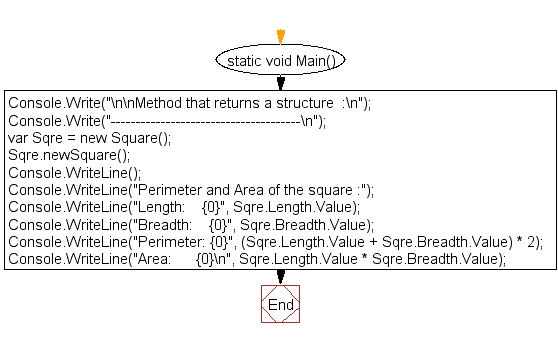﻿ C# Sharp Exercises: Method that returns a structure - w3resource# C# Sharp Exercises: Method that returns a structure

## C# Sharp STRUCTURE: Exercise-10 with Solution

Write a program in C# Sharp to implement a method that returns a structure including calling the method and using its value.

Sample Solution:-

C# Sharp Code:

``````using System;

public struct sampStru
{
private double val;
public double Value
{
get { return val; }
set { val = value; }
}
{
}
}
public struct Square
{
sampStru ln;
sampStru ht;

public sampStru Length
{
get { return ln; }
set { ln = value; }
}
{
get { return ht; }
set { ht = value; }
}
public void newSquare()
{
sampStru rct = new sampStru();

Console.WriteLine("\nInput the dimensions of the Square( equal length and breadth ) : ");
ln = sqrLength();
}
public sampStru sqrLength()
{
sampStru rct = new sampStru();

Console.Write("length : ");
return rct;
}
}
public class strucExer10
{
static void Main()
{
Console.Write("\n\nMethod that returns a structure  :\n");
Console.Write("--------------------------------------\n");
var Sqre = new Square();
Sqre.newSquare();
Console.WriteLine();
Console.WriteLine("Perimeter and Area of the square :");
Console.WriteLine("Length:    {0}", Sqre.Length.Value);
Console.WriteLine("Perimeter: {0}", (Sqre.Length.Value + Sqre.Breadth.Value) * 2);
}
}
```
```

Sample Output:

```Method that returns a structure  :
--------------------------------------
Input the dimensions of the Square( equal length and breadth ) :
length : 10

Perimeter and Area of the square :
Length:    10
Perimeter: 60
Area:      200
```

Flowchart:C# Sharp Code Editor: